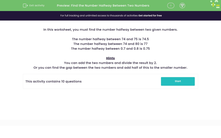# Find the Number Halfway Between Two Numbers

In this worksheet, students will find the number halfway between two given numbers.Key stage:  KS 3

Curriculum topic:   Number

Curriculum subtopic:   Use Place Value for All Numbers

Difficulty level:#### Worksheet Overview

In this activity, we will be finding the number halfway between two given numbers.

The number halfway between 74 and 75 is 74.5

The number halfway between 74 and 80 is 77

The number halfway between 0.7 and 0.8 is 0.75

How to work it out

You can add the two numbers and then divide the result by 2.

Or you can find the gap between the two numbers and add half of this to the smaller number.

For example, what number is halfway between 87 and 91?

87 + 91 = 178 ÷ 2 = 89

The gap between 87 and 91 is: 91 - 87 = 4

Half of 4 is 2.

87 + 2 = 89

Both methods work, so you should do whichever one works best for you - aren't we kind?!### What is EdPlace?

We're your National Curriculum aligned online education content provider helping each child succeed in English, maths and science from year 1 to GCSE. With an EdPlace account you’ll be able to track and measure progress, helping each child achieve their best. We build confidence and attainment by personalising each child’s learning at a level that suits them.

Get started